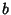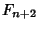## Lamé's Theorem

Ifis the smallest Integer for which there is a smaller Integersuch thatandgenerate a Euclidean Algorithm remainder sequence withsteps, thenis the Fibonacci Number. Furthermore, the number of steps in the Euclidean Algorithm never exceeds 5 times the number of digits in the smaller number.